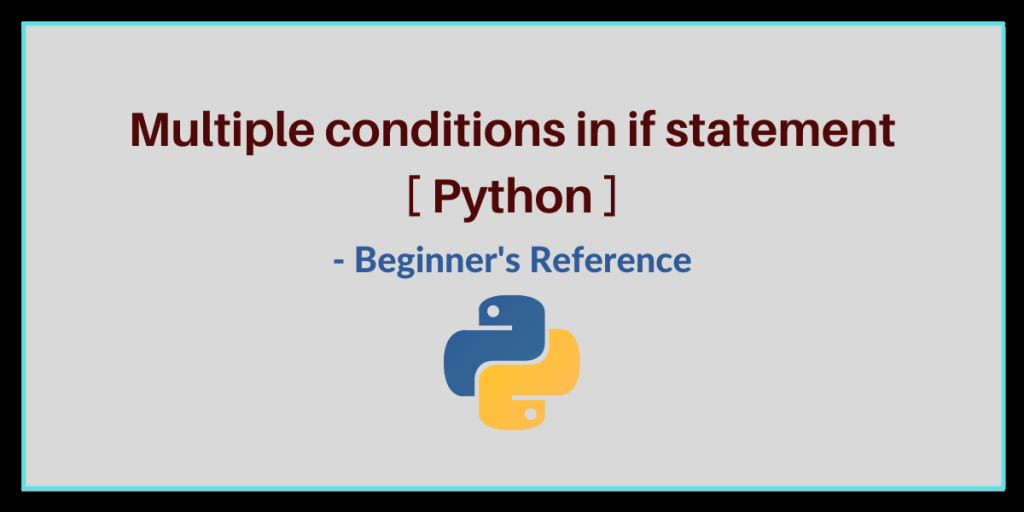# Multi-Conditional If Statement in Python [Explained]Hello learner! Today, we will understand how to implement multiple conditions in the ‘ if statement‘. By the end of this article, you will learn different cases of implementing the if-else condition. So let’s begin.

## What is the if statement in Python?

‘If’ statement is a conditional statement that is used to check whether a particular expression is true or not. The program control first checks the condition written with ‘ if ‘ and if the condition proves to be true, the if block is executed. Otherwise, the program control goes to the else block and executes it.

SYNTAX:

```if(condition) :
code block 1
else :
code block 2
```

Code block 1 executes if the condition is satisfied. If not, code block 2 is executed.

We all generally use a basic if statement, i.e., if statement with only one condition. This is used when we want to compare a variable with another variable or we want to check if a variable is true or not. for eg:

```num1 = int(input("Enter a number:")

if( num1 % 2 == 0 ):
print("The number is even!")
else:
print("The number is odd!")
```

Output:

```Enter a number: 37
The number is odd!
```

## How to use multiple conditions in an if statement?

Now, we will see how to use multiple conditions in an if statement. The syntax and example are explained below:

SYNTAX:

```if ((cond1) AND/OR (cond2)) :
code block 1
else :
code block 2
```

The multiple conditions can be used using AND or OR or BOTH in the single if statement.

### 1. Multiple conditions using ‘and’

AND condition is used when you want all the conditions to be satisfied. Take a look at the below example:

```age = int (input (" What is your age? "))
exp = int (input (" Enter your work experience in years: "))

if (age > 30 and age < 60) and (exp > 4):
Print (" You are hired! ")
else:
Print (" Sorry! you are not eligible :( ")
```

The above code uses AND condition which means every condition written must be true. Age must be between 30 to 60 and experience should be more than 4 years, then only you’ll be hired.

```Output:
Enter your work experience in years: 6
You are hired!

Enter your work experience in years: 5
Sorry! you are not eligible :(

```

### 2. Multiple conditions using ‘or’

OR condition is used when you want at least one condition to be satisfied. Let’s take a look at an example:

```num1 = int(input("Enter any number : "))
rem = num1 % 10

if (rem == 0 ) or ( rem == 5 ) :
print( "{} is divisible by 5 ".format(num1))
else :
print(" {} is not divisible by 5".format(num1))
```

The above code checks whether the entered number is divisible by 5 or not. For that, it first finds out the last digit of the number by finding out the remainder when divided by 10 (Using modulo 10) and if the remainder is equal to 0 or 5, it prints that the number is divisible by 5. If not, it prints that the number is not divisible by 5.

```OUTPUT :

Enter any number : 90
90 is divisible by 5

Enter any number : 27
27 is not divisible by 5

Enter any number : 15
15 is divisible by 5
```

## Conclusion

So, this was how we can use multiple conditions in an if statement. Do try out different combinations of if-else conditions and feel free to drop questions below if any!

Thank you! 🙂